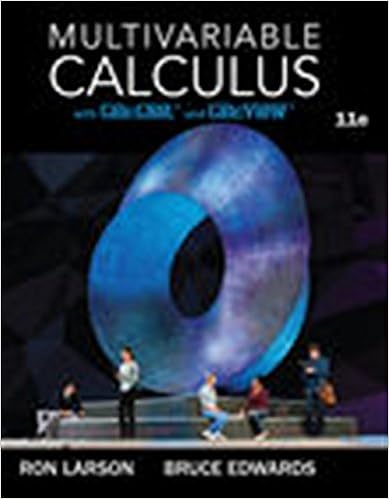# 26 fig 2 sample tape and demonstration of falling bob

• Lab Report
• 86
• 78% (9) 7 out of 9 people found this document helpful

This preview shows page 32 - 38 out of 86 pages.

##### We have textbook solutions for you!
The document you are viewing contains questions related to this textbook.The document you are viewing contains questions related to this textbook.
Chapter 15 / Exercise 23
Multivariable Calculus
LarsonExpert Verified
26
##### We have textbook solutions for you!
The document you are viewing contains questions related to this textbook.The document you are viewing contains questions related to this textbook.
Chapter 15 / Exercise 23
Multivariable Calculus
LarsonExpert Verified
Fig. 2 - Sample tape and demonstration of falling bobWhen you obtain a tape, inspect it and draw a small circle around each mark made by the spark apparatus to help with the identification of the position marks. You will obtain the acceleration ofgravity, g, by two methods. The difference in the methods is in the analysis of the data on the tape.Method I:1.Choose a starting point and from that point on, label your points, 1, 2, 3 . . . n.2.Obtain the distance ÄS in cm between two successive points. For example, assume the distance between points 3 and 4 is: 4.52cm. Thus, ÄS=4.52cm.3.Obtain the average velocity over each of these distances. Note that the time interval Ät between two successive points is s4. Obtain the successive changes in average velocities ÄV then use these changes to compute theacceleration for each particular change..27
Fig. 3 - Sample graph of V vs t5. Tabulate your data as follows:nÄS[cm]t= n Ä[s][cm/s]ÄV[cm/s][cm/s2]11/6022/6033/60......nn/60Note: 6.Obtain g by taking the average of the values of a on the 6thcolumn of your table. State g and the% differenceof your result.Method II:1.Plot a graph of velocity V versus time t; the independent variable should be plotted on theabscissa and the dependent variable along the ordinate. 2.Determine g from the slope of the graph, the units should be cm/s2.t28
3.Convert your value of g from cm/s2to m/s2. Compute the % difference.4.Estimate errors of g and compare your results with the theoretical value of g = 9.80 m/s2.Questions:1.Does any part of the experiment show that all bodies fall with constant acceleration?2.What is the significance of the constants in the equation relating V and t you plotted?3.In relation to your experimental data:3a. Why doesn't the graph of V versus t (Method 2) go through the origin?3b. At what time did the body start to fall?3c. With what velocity did the body start to fall?3d. Can you determine the position of the bob when it started to fall?4.What physical significance does negative time have in your equation relating V and t?5.In terms of a V vs t graph:5a. What would be the effect on your graph if there is a change in the time interval betweensparks?5b. What would be the effect if we had used a body with twice the original mass of the body todo the experiment?6.In regards to the two methods used in this experiment:6a. What are the advantages in terms of analysis by Method 2 as compared to Method 1?6b. Which method do you feel is the “best?” 6c.What are the advantages (or disadvantages) in Method 1 over that Method 2? Support youranswer.29
30
Fig. 1 - Set-upHORIZONTAL PROJECTILE MOTIONApparatus: SOne long steel poleSOne short steel poleSOne table clampSOne small V-groove clampSOne right angle clampSLaunching trackSSmall steel ballSLevel and plumb bobSCarbon paperS11" x 17" white paper and masking tapeSMeter stick and rulerIntroduction:For a projectile near the surface of the earth the position of a particle in a trajectory is broken up intoits Xand Ycoordinates in the plane of the trajectory. Thus, we examine the most general vector
•••